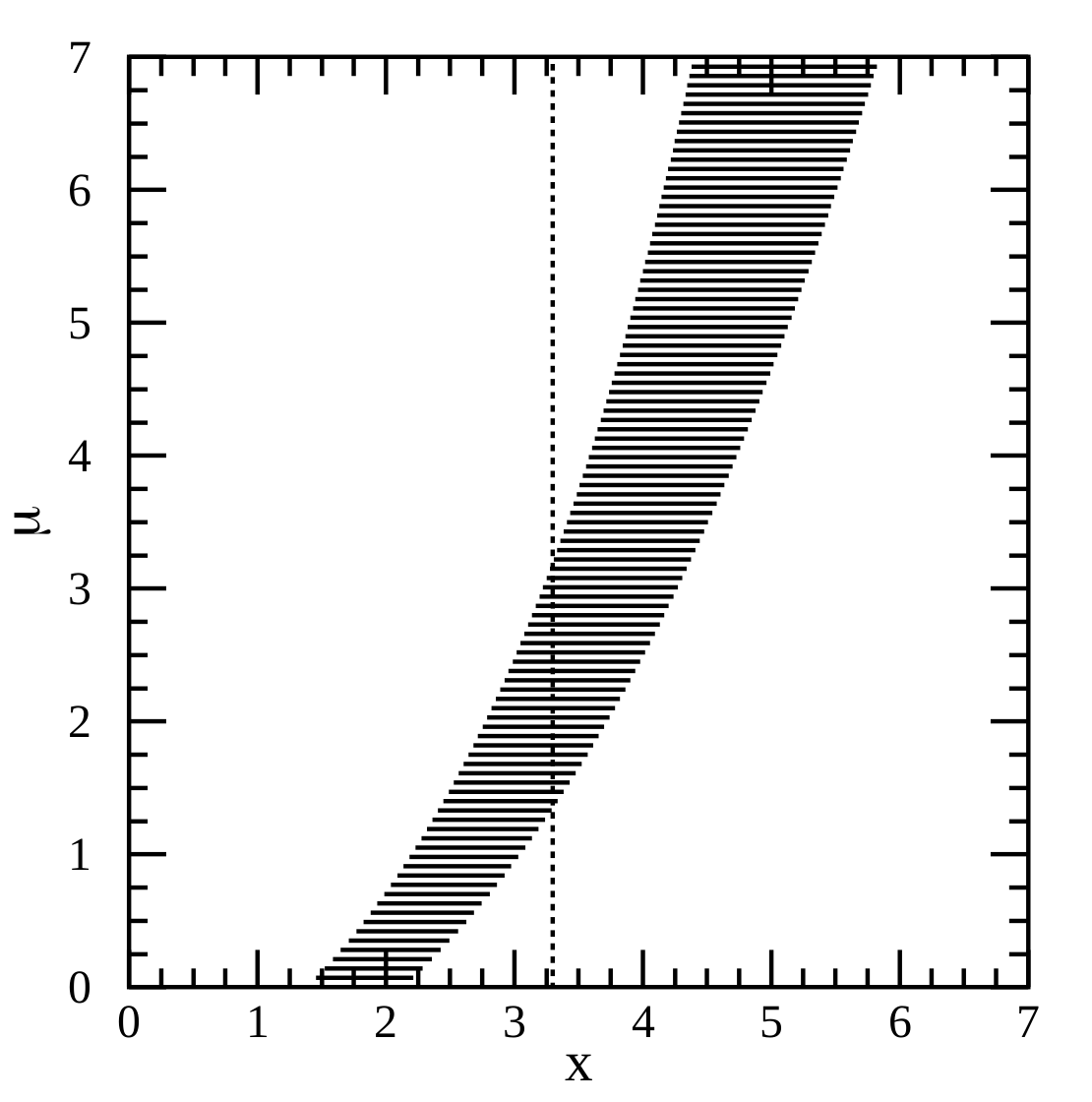# New solutions for Parker sperm competition model

Parker et al. (2013) created a general model for sperm allocation under a trade-off between male investment of resources $$R$$ into pre-copulatory effort (e.g. search time) $$T$$ versus post-copulatory effort (e.g. ejaculate) $$U$$. Their model is interesting because it encompasses a range of different scenarios of female remating and the type of competition between males. For female remating scenarios, the risk model has females mating … Continue reading New solutions for Parker sperm competition model# Playing with a new model for fugitive coexistence

I recently read a paper by Kawecki (2017), which presents a new mechanism for something analogous to fugitive coexistence. The paper has a really great literature overview, which I won’t be able to do justice here. In short, fugitive coexistence is when an inferior species persists on a patchy landscape by being a better coloniser: when a local extinction occurs, they are quicker to arrive … Continue reading Playing with a new model for fugitive coexistence

# Fixation probability of birth-death process

The goal is to understand where Eq. 2 of the Supplementary section of Sigmund et al. (2010) came from. We are considering a finite population within which individuals are pursuing different game-theoretic strategies. At each timestep, a pair of individuals is chosen at random, and they engage in a social learning process, where individual $$i$$ will adopt the strategy of individual $$j$$ according … Continue reading Fixation probability of birth-death process# Moments for a bivariate beta distribution

A common choice for a probability distribution of a probability is the beta distribution. It has the required support between 0 and 1, and with its two parameters we can obtain a pretty wide qualitative range for the probability density function. What should we do if we want to create correlated probabilities? We might look for some kind of multivariate generalisation of the beta distribution, … Continue reading Moments for a bivariate beta distribution# The method of confidence belts illustrated

What is a confidence interval, really? We all learnt in undergrad how to find CIs for a standard distribution, but plugging numbers into equations never gave me a deep intuition for what was really going on. A worded definition is probably more helpful. Paraphrasing a bit from Wikipedia, we can think of the meaning of the confidence interval in terms of the procedure that we … Continue reading The method of confidence belts illustrated

# Estimating undetected extinctions

The purpose of this blog post is to give a simplified account of how the Chisholm et al. (2016) method works for estimating undetected extinctions. To estimate the historical extinction rate within a taxonomic group, a naive approach would be to divide the number of species known to be extinct by the total number of species. However, this does not account for the historical process … Continue reading Estimating undetected extinctions

# Understanding the neigbour-modulated inclusive fitness approach

The goal is to understand Equation 4.2 of Rodrigues and Kokko (2016). To understand the technique, I read through Taylor et al. (2007), and Taylor and Frank (1996), specifically examples 4, 4a and 4b. Very briefly, we begin with a matrix $$A = [w_{i,j} ]$$ whose elements represent the genetic contribution of class $$j$$ to class $$i$$. Then for … Continue reading Understanding the neigbour-modulated inclusive fitness approach

# Some notes on the Principle of Indifference

A classical statement of the Principle of Indifference (PI) is as follows (p. 45 Keynes, 1921): if there is no known reason for predicating of our subject one rather than another of several alternatives, then relatively to such knowledge the assertions of each of these alternatives have an equal probability. Thus equal probabilities must be assigned to each of several arguments, if there is an … Continue reading Some notes on the Principle of Indifference

# Fibonacci numbers and alternating signs in species responses to press perturbation in a food chain

In a paper from 2001, Dambacher and Rossignol made a curious observation: Fibonacci numbers appear in the adjoint and absolute feedback matrices that result from a weighted-predictions matrix type analysis (Dambacher et al. 2003) on food chains. The weighted-predictions matrix analysis is a way of predicting how species in a food web will respond to a the press perturbation of one of the species, so … Continue reading Fibonacci numbers and alternating signs in species responses to press perturbation in a food chain

# The Principle of Indifference is actually two principles in one

In a previous post, I wrote about the philosophical problems caused by the Principle of Indifference. The problems are illustrated with a variety of thought-experiments that create paradoxes, such as Bertrand’s paradox. I also discussed how a problem in ecological modelling for conservation decision-making seems closely related to this philosophical problem. When I realised this connection, it seemed to me that, in order to solve … Continue reading The Principle of Indifference is actually two principles in one